#1

## [R語言]資料分析讀書心得03---Geometric Objects

``````library(tidyverse)
``````
``````ggplot(data=mpg)+
geom_point(mapping=aes(x=displ,y=hwy))
``````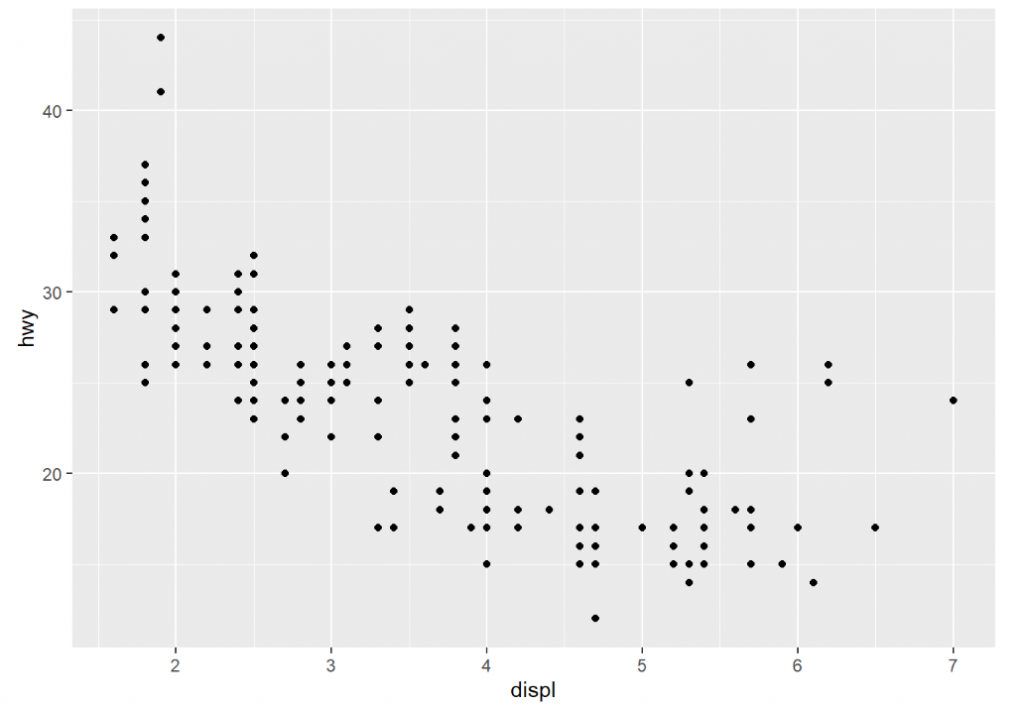``````ggplot(data=mpg)+
geom_smooth(mapping=aes(x=displ,y=hwy))
``````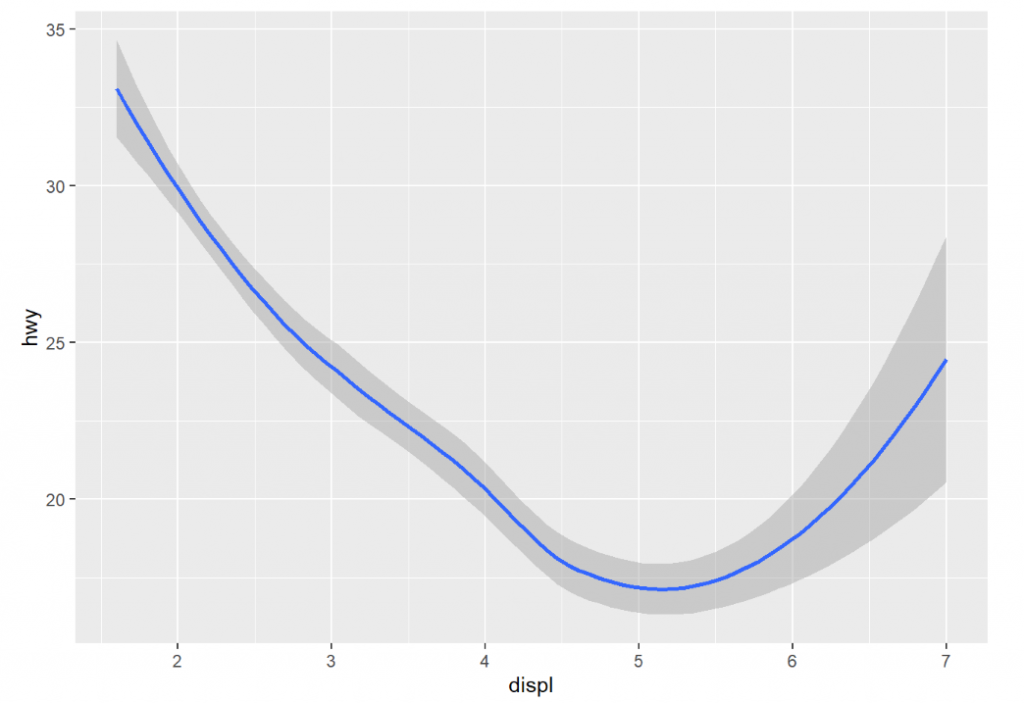``````ggplot(data=mpg)+
geom_smooth(mapping=aes(x=displ,y=hwy,linetype=drv))
``````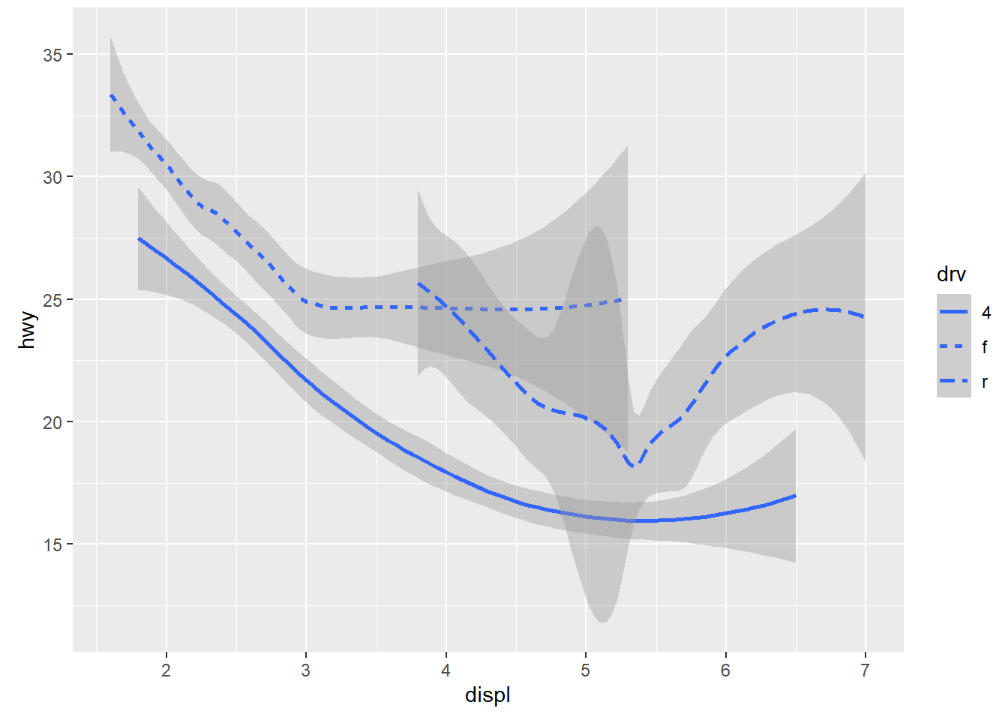x,y,alpha,color,fill,group,linetype,size,weight,ymax,ymin

``````ggplot(data=mpg)+
geom_smooth(mapping=aes(x=displ,y=hwy,group=drv))
``````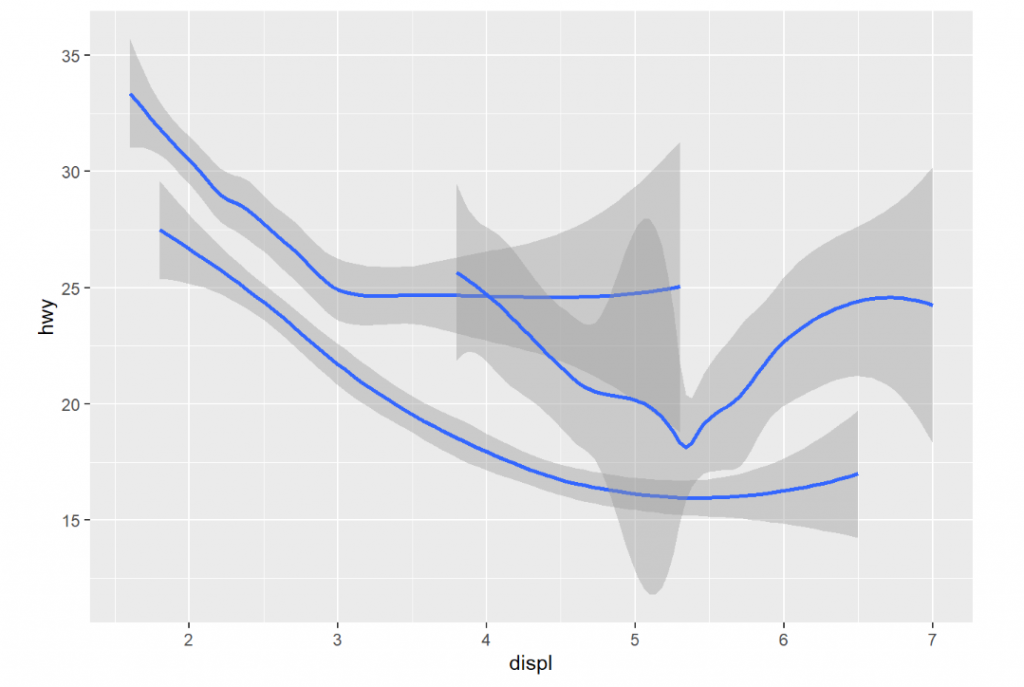``````ggplot(data=mpg)+
geom_smooth(mapping=aes(x=displ,y=hwy,color=drv))
``````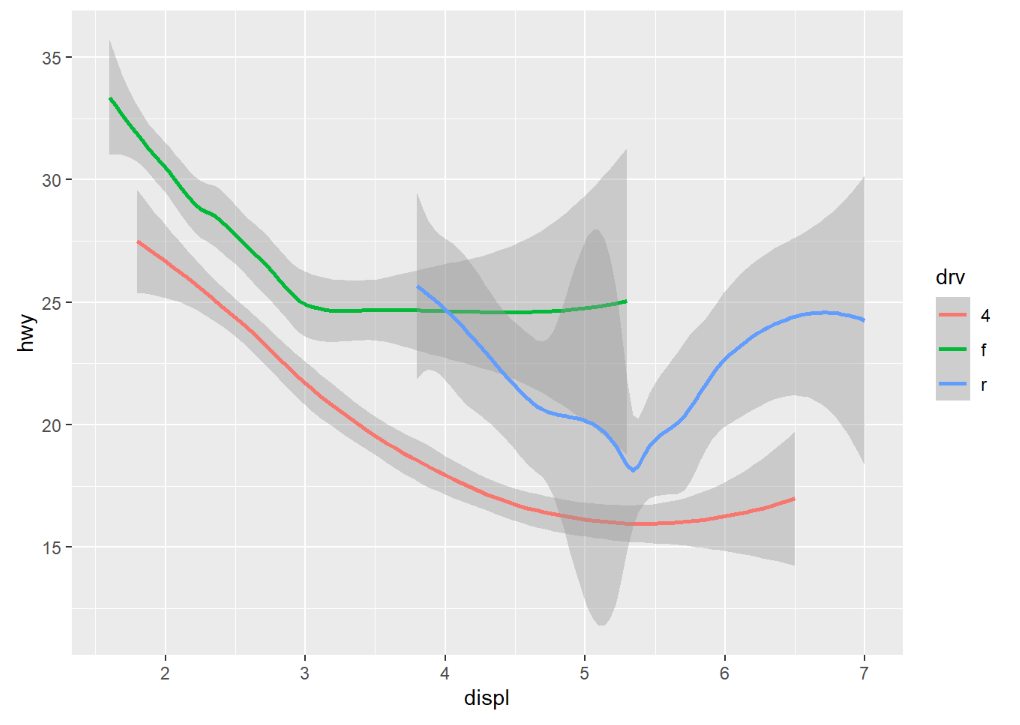``````ggplot(data=mpg)+
geom_smooth(mapping=aes(x=displ,y=hwy))+
geom_point(mapping=aes(x=displ,y=hwy))
``````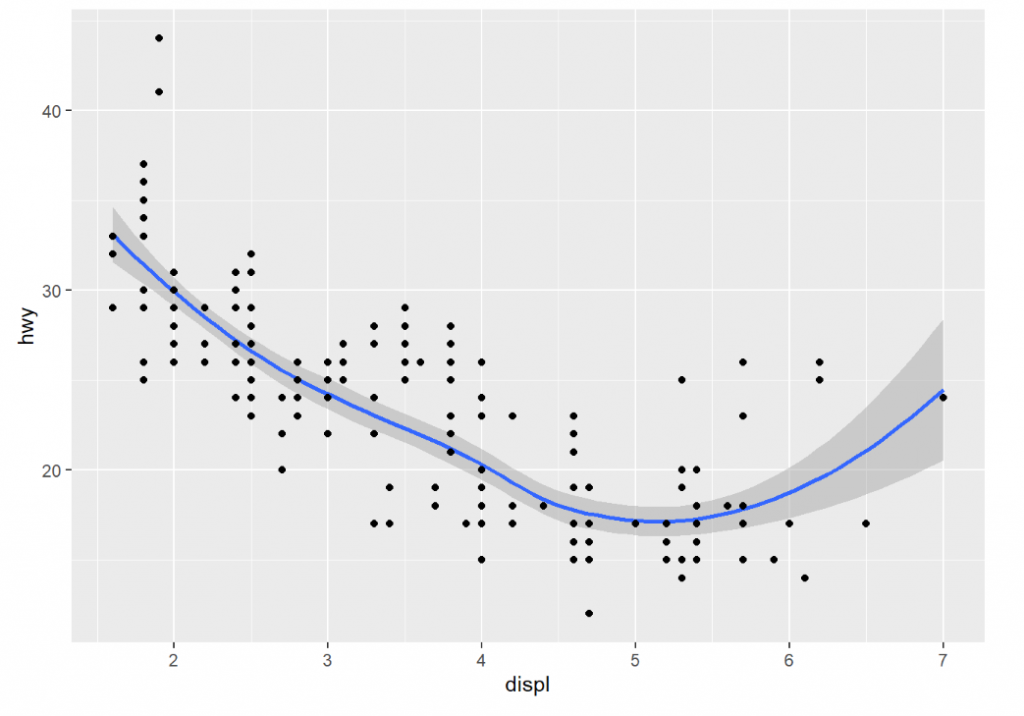``````ggplot(data=mpg,mapping=aes(x=displ,y=hwy))+
geom_smooth()+
geom_point()
``````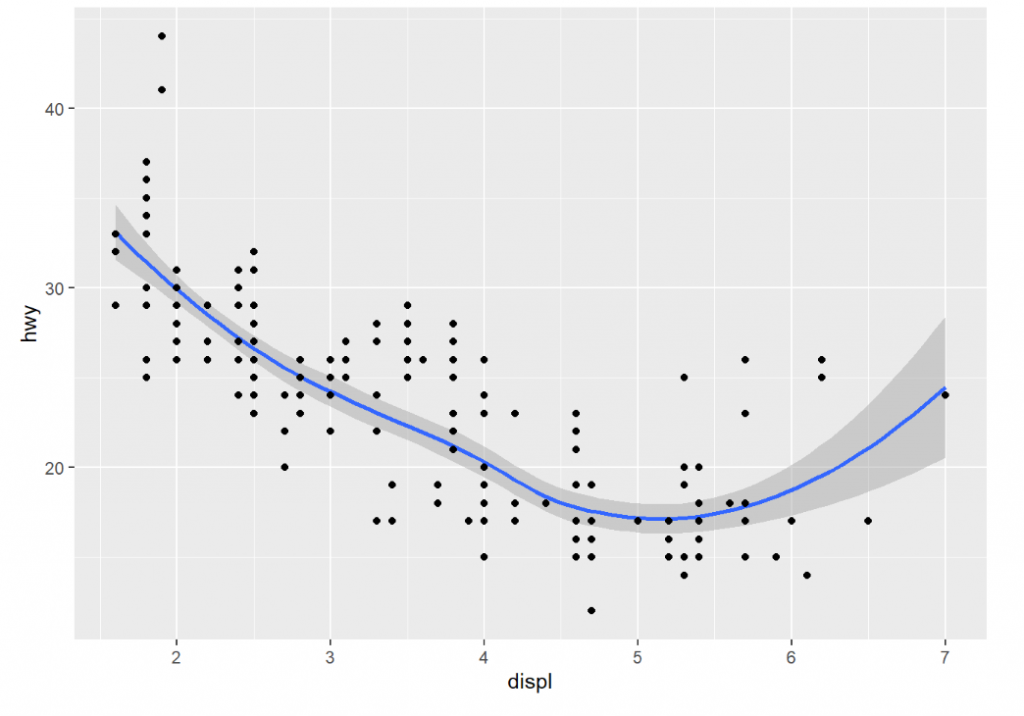``````ggplot(data=mpg,mapping=aes(x=displ,y=hwy))+
geom_smooth()+
geom_point(mapping=aes(color=class))
``````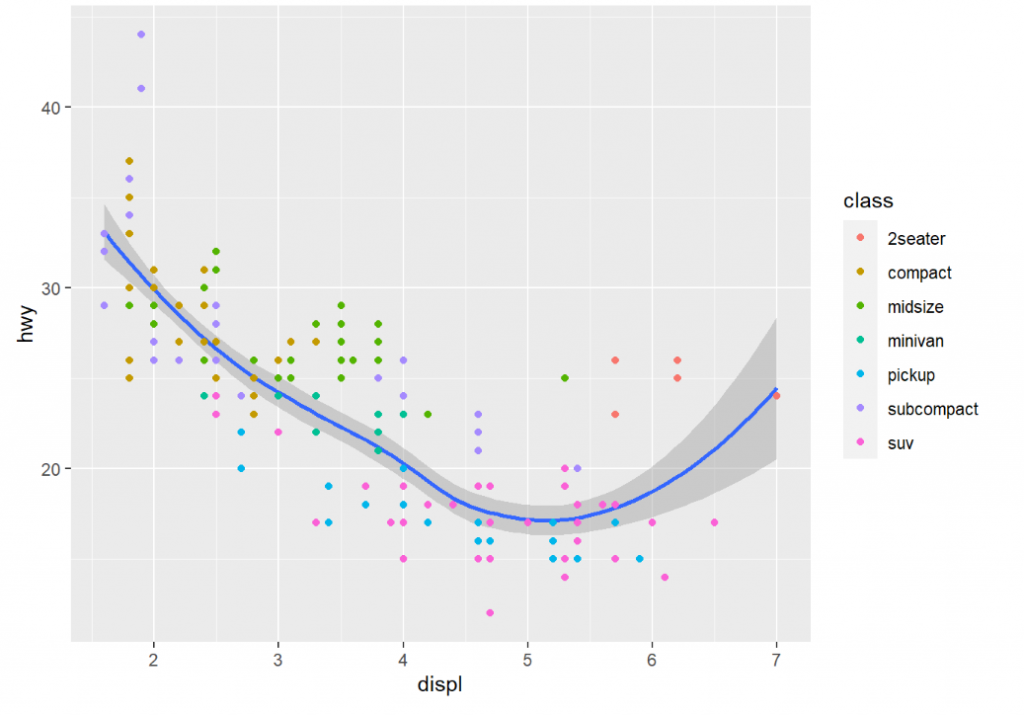``````ggplot(data=mpg,mapping=aes(x=displ,y=hwy))+
geom_smooth(
data=filter(mpg,class=="subcompact")
)+
geom_point(mapping=aes(color=class))
``````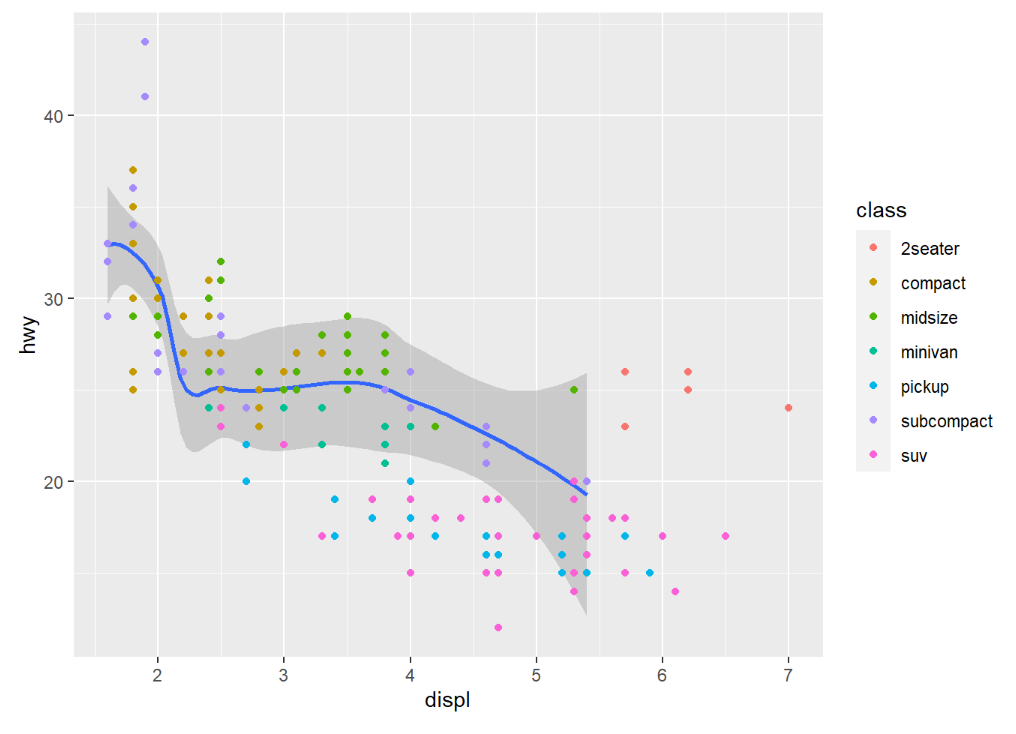``````ggplot(data=mpg,mapping=aes(x=displ,y=hwy))+
geom_smooth(
data=filter(mpg,class=="subcompact") ,se=FALSE
)+
geom_point(mapping=aes(color=class) ,show.legend = FALSE)
``````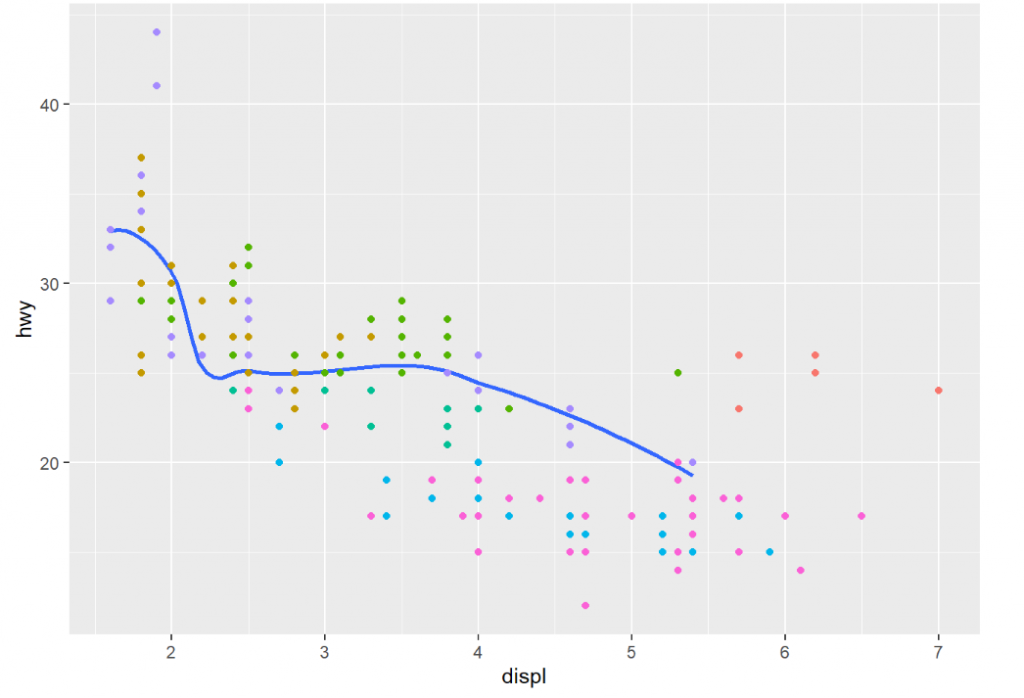ggplot2 中的 geoms 超過三十種，但是操作方式與 geom_pointgeom_smooth 大致上相似，所以可以根據你想繪製的圖表去找到適合的 geoms ，再加上我們可以同時展示兩種以上的 geoms ，那繪製出精美的圖片似乎也不是問題了。這次到介紹到這裡，謝謝大家。